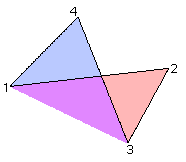Understanding the midpoint polygons of self-intersecting quadrilaterals requires use of the oriented, or algebraic, area. For a triangular region, this means that the area is positive if we order the points in such a way that we move counter-clockwise around the region, and negative if we move clockwise.Figure 3. The triagle on the left is oriented in the counter-clockwise direction and so has positive area; the triangle on the right has negative area.

This actually gives us the oriented area for any planar polygon; we just subdivide it into triangles, calculate their areas, and sum them up. In fact, it doesn't even matter if parts of our triangles lie outside the region, as in the quadrilateral below: